Next: 4.2.4 Discretization of the Up: 4.2 Discretization of the Previous: 4.2.2 Discretization of the

## 4.2.3 Discretization of the Vacancy Balance Equation

The combination of (3.74) with (3.75) and (3.76) yields the vacancy balance equation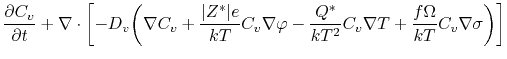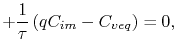(4.41)

for which the weak formulation of the form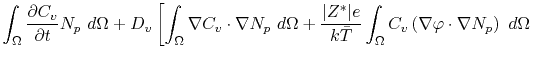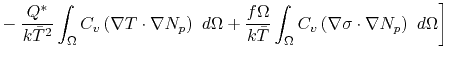(4.42)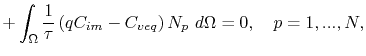is obtained under the assumption of a Neumann boundary condition. Applying the electric potential and the temperature discretization, (4.29) and (4.36), respectively, together with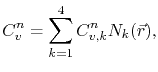(4.43)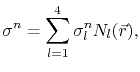(4.44)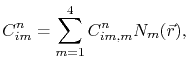(4.45)

and the backward Euler time discretization, the vacancy balance equation discretized in a single element is given by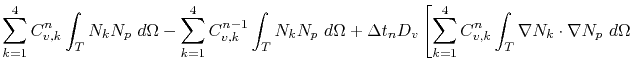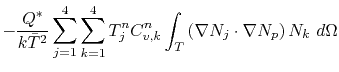(4.46)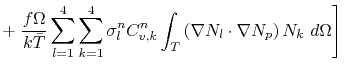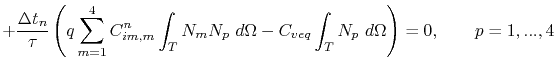under the assumption that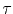,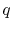, and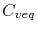are constant inside an element.

Using the shorthand notation (4.31), (4.38), (4.39), and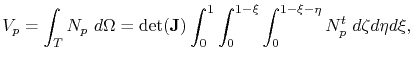(4.47)

(4.46) is written as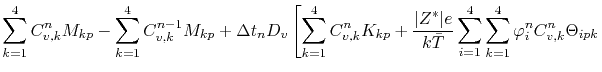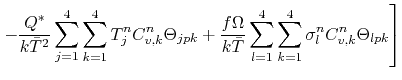(4.48)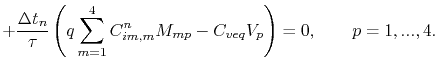In the above derivation the vacancy diffusivity is treated as a scalar diffusion coefficient. In order to take into account the anisotropy of diffusivity due to the mechanical stress, as presented in Section 3.2.2, a diffusivity tensor must be applied. This requires a slight modification of (4.48) to(4.49)where the diffusivity tensor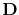is now incorporated into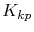and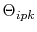, given by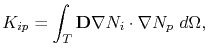(4.50)

and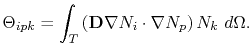(4.51)

At material interfaces and grain boundaries the trapped vacancy concentration is governed by (3.76), rewritten here as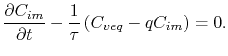(4.52)

The finite element formulation of this equation follows the same procedure described above, which yields the discretization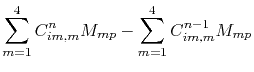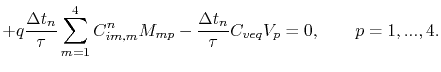(4.53)Next: 4.2.4 Discretization of the Up: 4.2 Discretization of the Previous: 4.2.2 Discretization of the

R. L. de Orio: Electromigration Modeling and Simulation